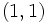# Equivalence of definitions of subgroup

This article gives a proof/explanation of the equivalence of multiple definitions for the term subgroup
View a complete list of pages giving proofs of equivalence of definitions

## The two definitions we have to prove as equivalent

### Definition in terms of closure under binary operation

This definition of subgroup corresponds to the textbook definition of group.

Let$G$ be a group. A subset$H$ of$G$ is termed a subgroup if the following two conditions hold:

• Whenever$a,b$ belong to$H$, the product$ab$ belongs to$H$.
• With this induced multiplication,$H$ becomes a group in its own right (i.e., it has an identity element, and every element has a two-sided inverse).

### The universal algebraic definition

This definition of subgroup corresponds to the universal algebraic definition of group.

Let$G$ be a group. A subset$H$ of$G$ is termed a subgroup if all the three conditions below are satisfied:

• Whenever$a, b$ belong to$H$, so does$ab$ (here$ab$ denotes the product of the two elements)
• Whenever$a$ belongs to$H$, so does$a^{-1}$ (the multiplicative inverse of$a$)
•$e$ belongs to$H$ (where$e$ denotes the identity element)

## Generalizations

The result fails to generalize to monoids. In other words, a subset of a monoid that is closed under multiplication may contain a neutral element (identity element) that is not equal to the neutral element for the whole monoid. Two counterexamples are below.

### 0 and 1 example

Let$G$ be the monoid$\{ 0, 1 \}$ with usual multiplication of real numbers. Let$H$ be the subset$\{ 0 \}$. Then,$H$ is a monoid in its own right with the multiplication inherited from$G$ and has identity element 0. However, it does not contain the identity element 1 of$G$.

### Direct product example

1.$G$ is the monoid of ordered pairs of integers, under coordinate-wise multiplication.
2.$H$ is the subset comprising pairs of the form$(a,0)$.

Then, the identity element in$G$ is$(1,1)$ while that in$H$ is$(1,0)$.

The problem here is the lack of cancellativity of elements of the form$(a,0)$.

## Proof

The proof relies on showing the following two things:

1. If a nonempty subset of the group is closed under the binary operation and contains an identity element for that binary operation, then the identity element of the subgroup must be equal to that of the group.
2. If every element has an inverse in the subset, then that inverse must equal its inverse in the whole group.

### Proof that identity elements are equal

Given: A group$G$ with identity element$e$, a nonempty subset$H$ with$f \in H$ such that$a * f = f * a = a$ for all$a \in H$

To prove:$f = e$

Proof: In a group, every element is invertible, hence every element is cancellative. Pick any$a \in H$. Then:$a * f = a = a * e$

Since$a$ is cancellative, we get:$f = e$

as desired.

### Proof that the inverse operation in the subgroup is the same as in the whole group

We have shown that the identity element in the subgroup is the same as that in the whole group, so the condition for being a two-sided inverse in the subgroup is the same as that in the whole group. By Fact (2), the two-sided inverse in the whole group is unique, forcing the two operations to agree.

### Note on maintenance of associativity, identity, and inverses

This is a minor note about one way in which the textbook definition seems to be somewhat more stringent than the universal algebra definition. The textbook definition states that the multiplication restricted to the subset gives a group structure, and we know that this includes associativity. The universal algebra definition does not include any postulate about associativity in the subset. However, this is a non-issue, because associativity holds in the whole group, hence it also holds when we restrict variables to the subset.